# AI从入门到放弃：BP神经网络算法推导及代码实现笔记## ▌一. 前言：

【毒鸡汤】：算法这东西，读完之后的状态多半是 --> “我是谁，我在哪？” 没事的，吭哧吭哧学总能学会，毕竟还有千千万万个算法等着你。## ▌二. 科普：

• 生物上的神经元就是接收四面八方的刺激（输入），然后做出反应（输出），给它一点☀️就灿烂。

• 仿生嘛，于是喜欢放飞自我的 某些人 就提出了人工神经网络。一切的基础-->人工神经单元，看图：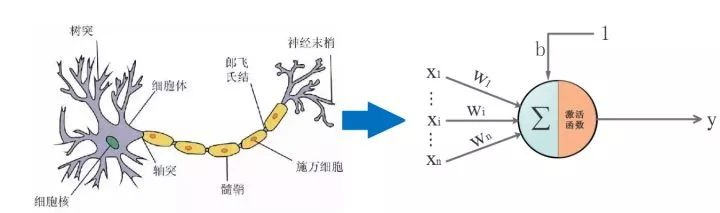## ▌三. 通往沙漠的入口: 神经元是什么，有什么用：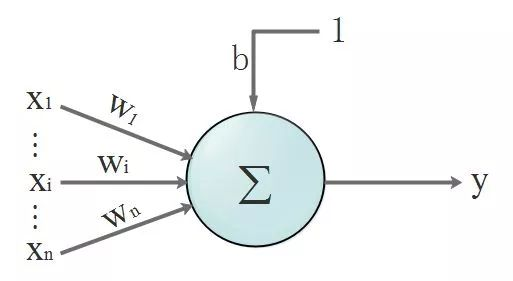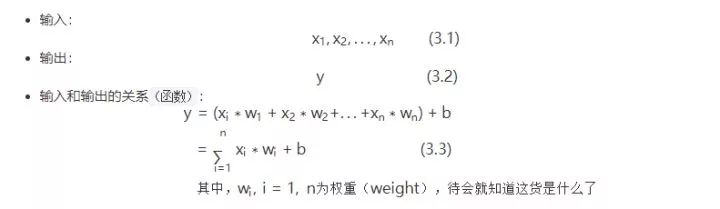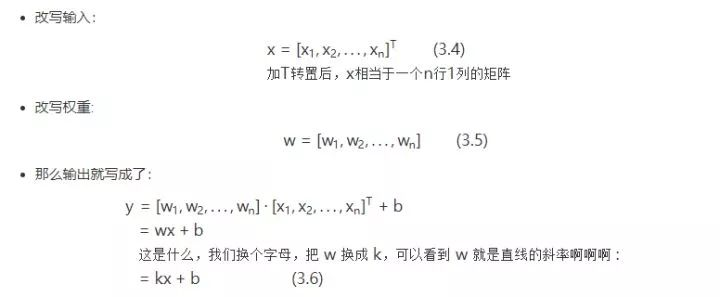• 一个神经元是什么：参照式（1.6），从函数图像角度看，这就是一根直线。

• 一个神经元有什么用：要说明用途就要给出一个应用场景：分类。一个神经元就是一条直线，相当于楚河汉界，可以把红棋绿棋分隔开，此时它就是个分类器。所以，在线性场景下，单个神经元能达到分类的作用，它总能学习到一条合适的直线，将两类元素区分出来。

http://t.cn/RBCoWof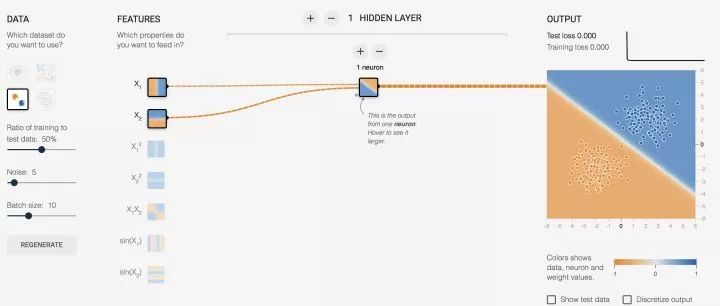• (x1,x2) 对于神经元的输入都是 x, 而对我们而言，这数据就是意义上的点的坐标，我们习惯写成 (x,y)。1. 假设，0代表红点，1代表蓝点（这些数据都是事先标定好的，在监督学习下，神经元会知道点是什么颜色并以这个已知结果作为标杆进行学习）

2. 当神经元输出小于等于 0 时，最终结果输出为 0，这是个红点

3. 当神经元输出大于 1 时，最终结果输出为 1，这是个蓝点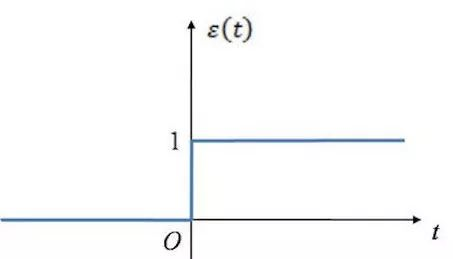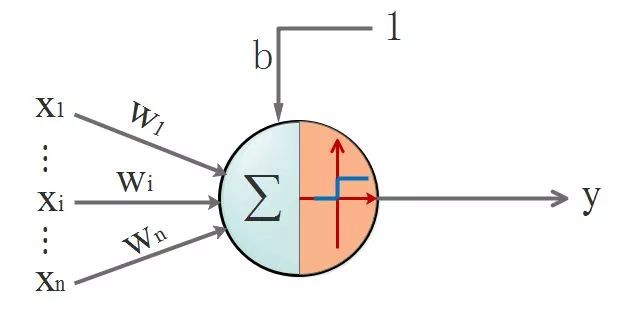## ▌四. 茫茫大漠第一步: 激活函数是什么，有什么用

• 非线性: 即导数不是常数,不然就退化成直线。对于一些画一条直线仍然无法分开的问题，非线性可以把直线掰弯，自从变弯以后，就能包罗万象了。

• 几乎处处可导：也就是具备“丝滑的特性”，不要应激过度，要做正常人。数学上，处处可导为后面降到的后向传播算法（BP算法）提供了核心条件

• 输出范围有限：一般是限定在[0,1]，有限的输出范围使得神经元对于一些比较大的输入也会比较稳定。

• 非饱和性：饱和就是指，当输入比较大的时候，输出几乎没变化了，那么会导致梯度消失！什么是梯度消失：就是你天天给女生送花，一开始妹纸还惊喜，到后来直接麻木没反应了。梯度消失带来的负面影响就是会限制了神经网络表达能力，词穷的感觉你有过么。sigmod，tanh函数都是软饱和的，阶跃函数是硬饱和。软是指输入趋于无穷大的时候输出无限接近上线，硬是指像阶跃函数那样，输入非0输出就已经始终都是上限值。数学表示我就懒得写了，传送门在此（https://www.cnblogs.com/rgvb178/p/6055213.html）,里面有写到。如果激活函数是饱和的，带来的缺陷就是系统迭代更新变慢，系统收敛就慢，当然这是可以有办法弥补的，一种方法是使用交叉熵函数作为损失函数，这里不多说。ReLU是非饱和的，亲测效果挺不错，所以这货最近挺火的。

• 单调性：即导数符号不变。导出要么一直大于0，要么一直小于0，不要上蹿下跳。导数符号不变，让神经网络训练容易收敛。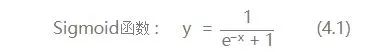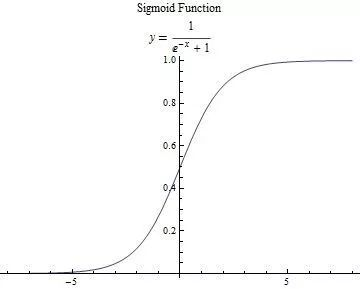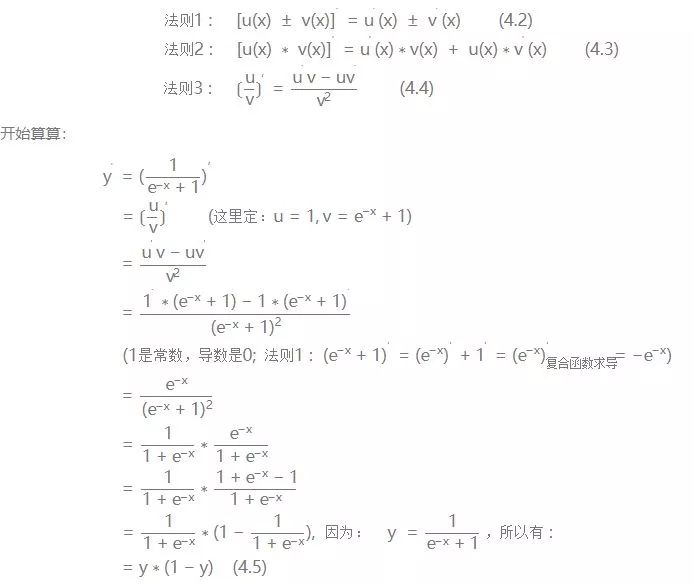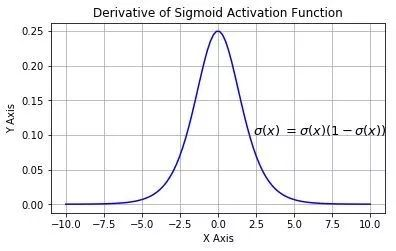## 1. 神经网络的结构

• 输入层：就是接收原始数据，然后往隐层送

• 输出层：神经网络的决策输出

• 隐藏层：该层可以说是神经网络的关键，相当于对数据做一次特征提取。隐藏层的意义，是把前一层的向量变成新的向量。就是坐标变换，说人话就是把数据做平移，旋转，伸缩，扭曲，让数据变得线性可分。可能这个不那么好理解，举个栗子：

https://cs.stanford.edu/people/karpathy/convnetjs//demo/classify2d.html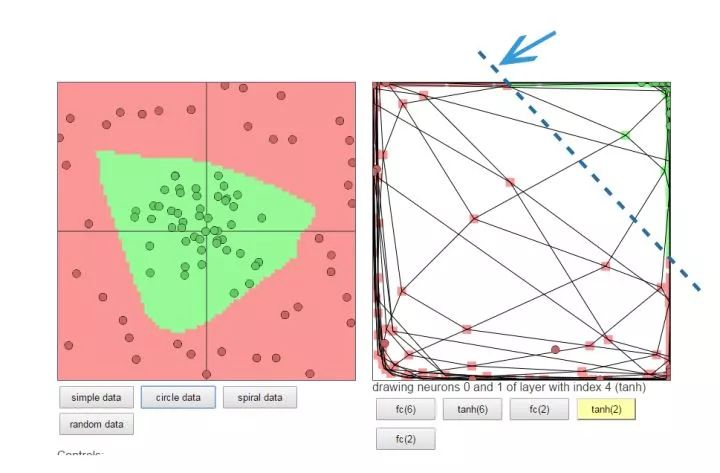## 2.正反向传播过程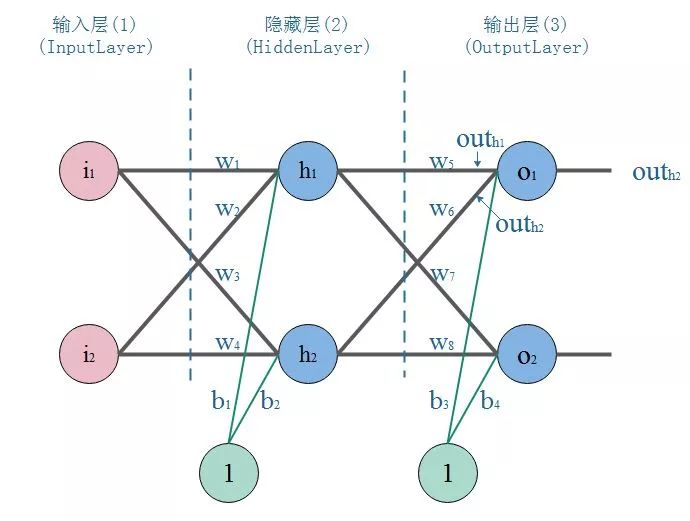• 正向传播：把点的坐标数据输入神经网络，然后开始一层一层的传播下去，直到输出层输出结果。

• 反向传播(BP)：就好比战士去靶场打靶，枪的摆放位置（输入），和靶心（期望的输出）是已知。战士（神经网络）一开始的时候是这样做的，随便开一枪（w，b参数初始化称随机值），观察结果（这时候相当于进行了一次正向传播）。然后发现，偏离靶心左边，应该往右点儿打。所以战士开始根据偏离靶心的距离（误差，也称损失）调整了射击方向往右一点（这时，完成了一次反向传播）

• 当完成了一次正反向传播，也就完成了一次神经网络的训练迭代，反复调整射击角度（反复迭代），误差越来越小，战士打得越来越准，神枪手模型也就诞生了。

## 3.BP算法推导和计算

• 参数初始化：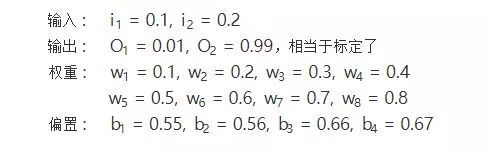• 正向传播：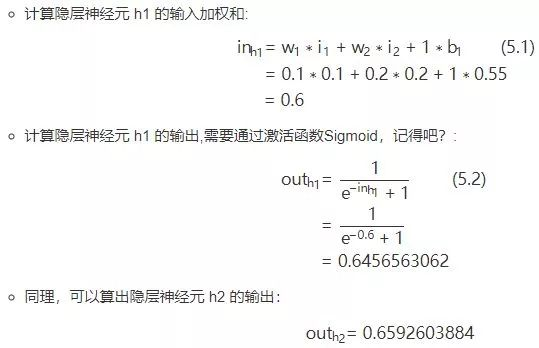2.隐层-->输出层：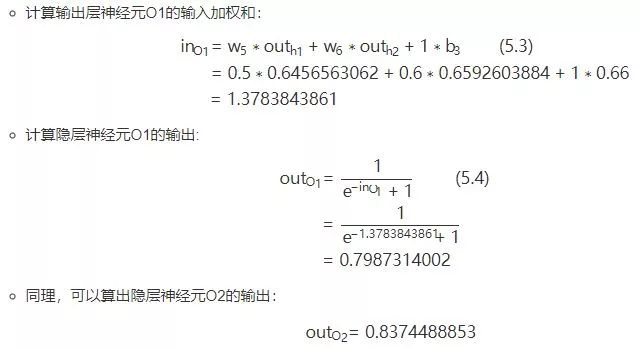• 反向传播：

1.计算输出误差：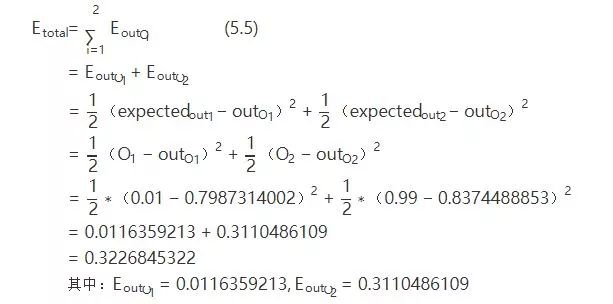PS: 这里我要说的是，用这个作为误差的计算，因为它简单，实际上用的时候效果不咋滴。如果激活函数是饱和的，带来的缺陷就是系统迭代更新变慢，系统收敛就慢，当然这是可以有办法弥补的，一种方法是使用交叉熵函数作为损失函数。2.隐层-->输出层的权值及偏置b的更新：

• 先放出链式求导法则：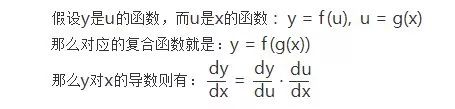• 以更新w5举例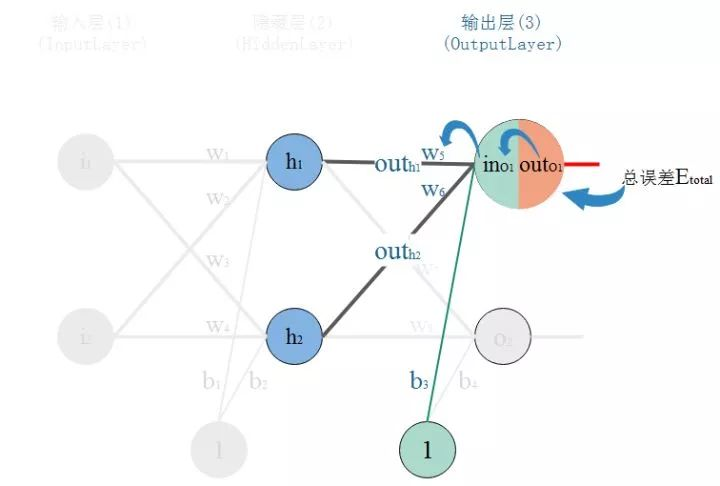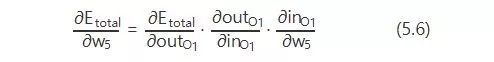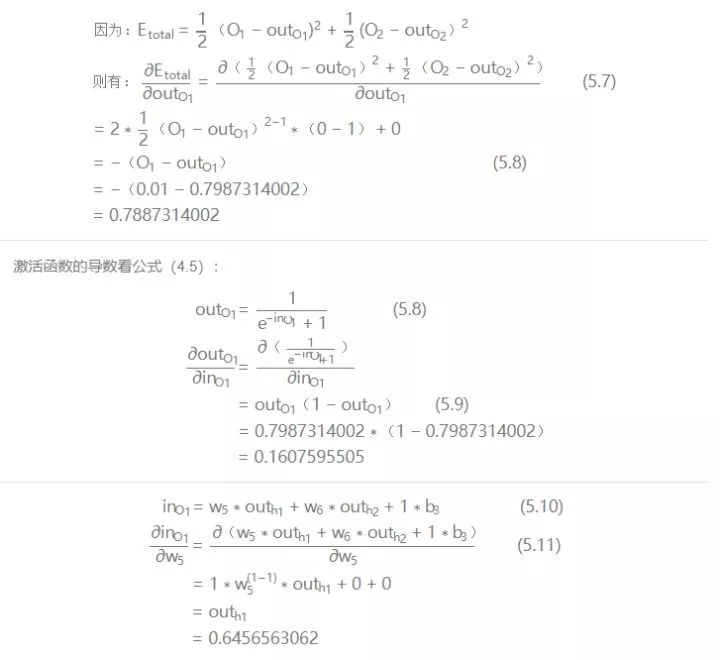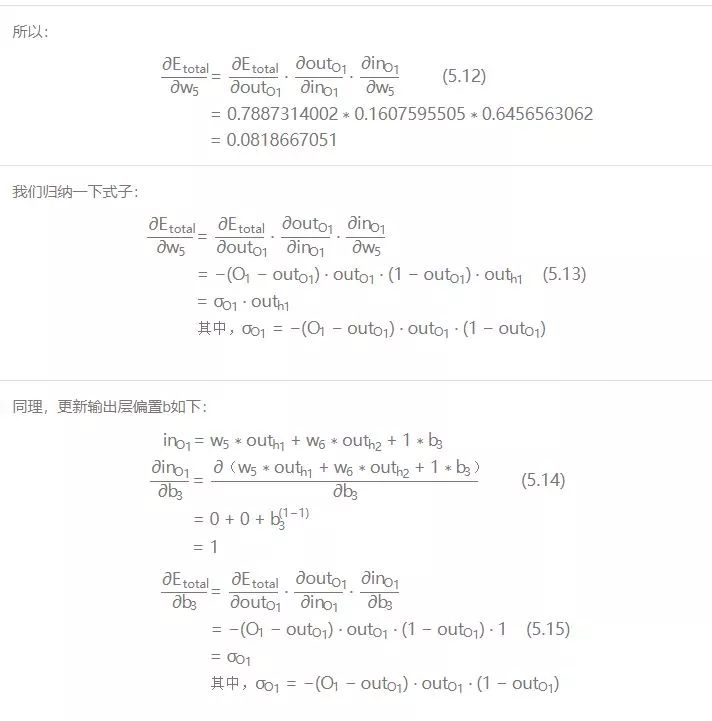xy轴是权值w平面，z轴是输出总误差。整个误差曲面可以看到两个明显的低点，显然右边最低，属于全局最优。而左边的是次低，从局部范围看，属于局部最优。而图中，在给定初始点的情况下，标出的两条抵达低点的路线，已经是很理想情况的梯度下降路径。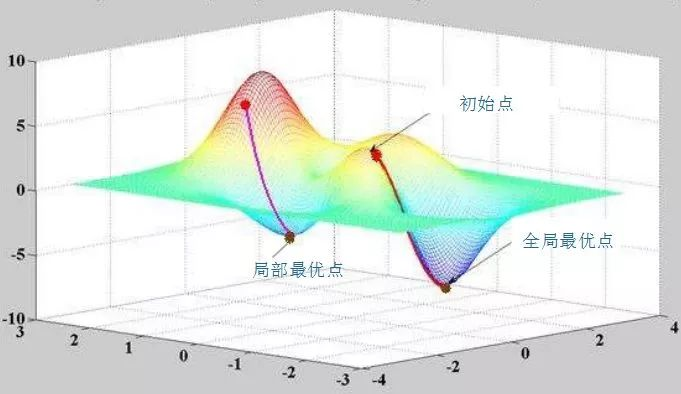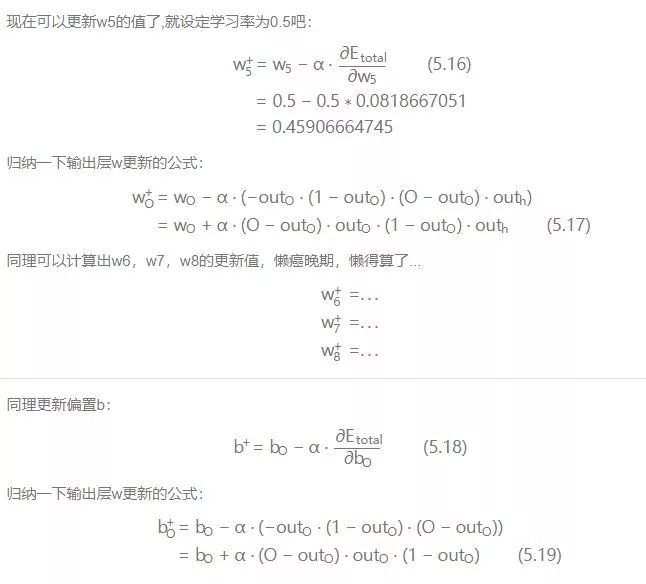3.输入层-->隐层的权值及偏置b更新：

• 以更新w1为例：
仔细观察，我们在求w5的更新，误差反向传递路径输出层-->隐层，即out(O1)-->in(O1)-->w5，总误差只有一根线能传回来。但是求w1时，误差反向传递路径是隐藏层-->输入层，但是隐藏层的神经元是有2根线的，所以总误差沿着2个路径回来，也就是说，计算偏导时，要分开来算。看图：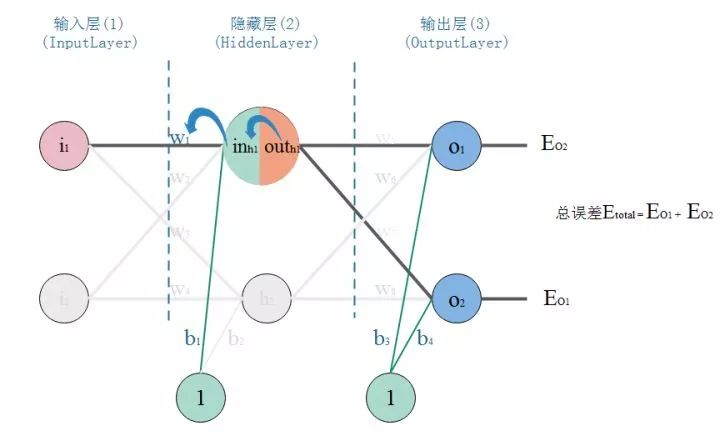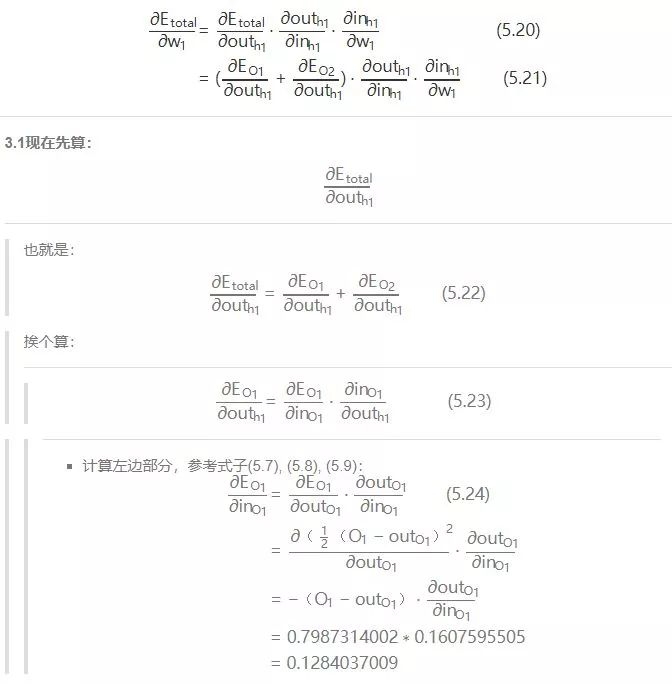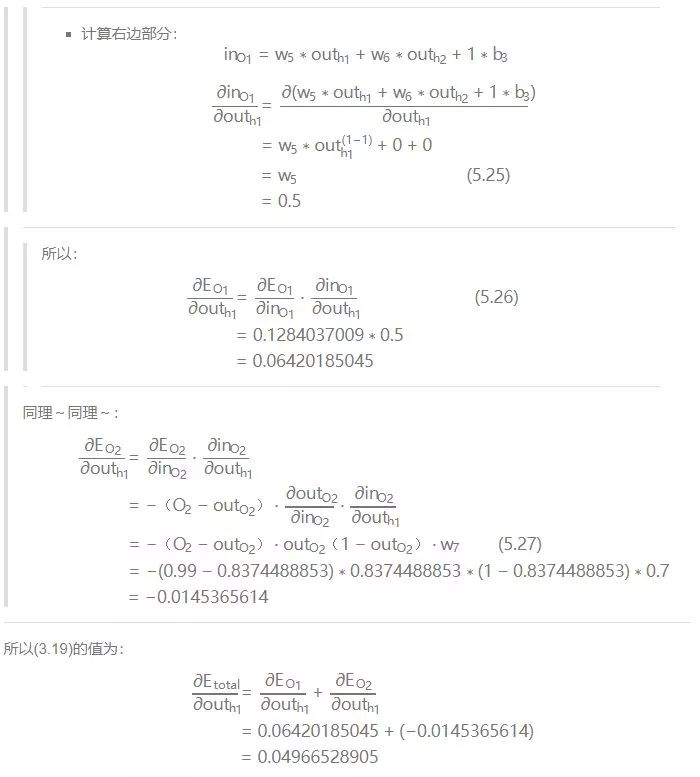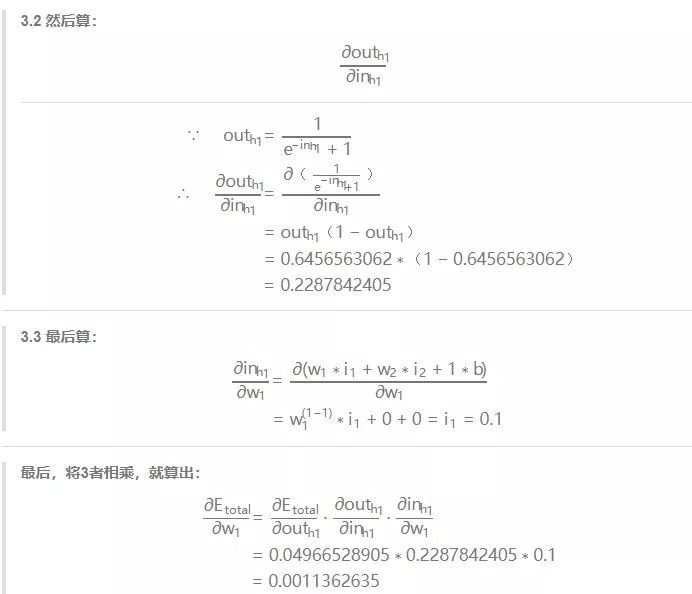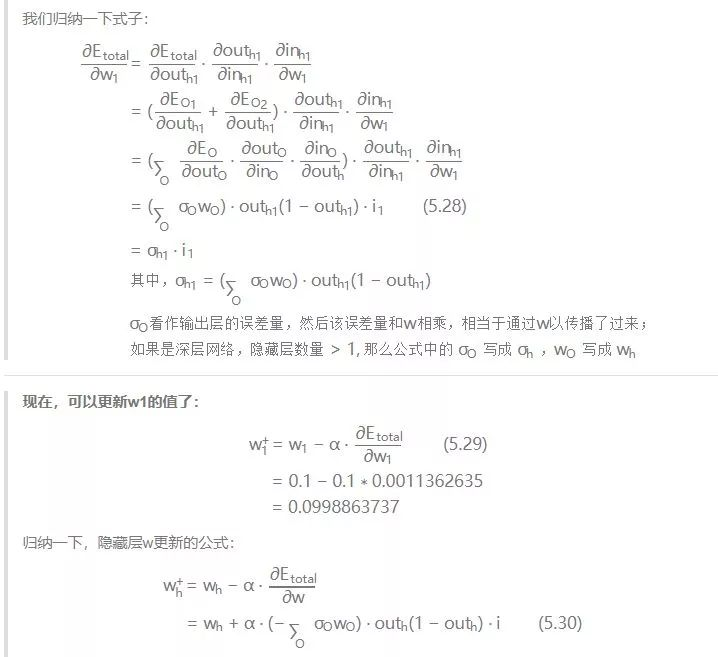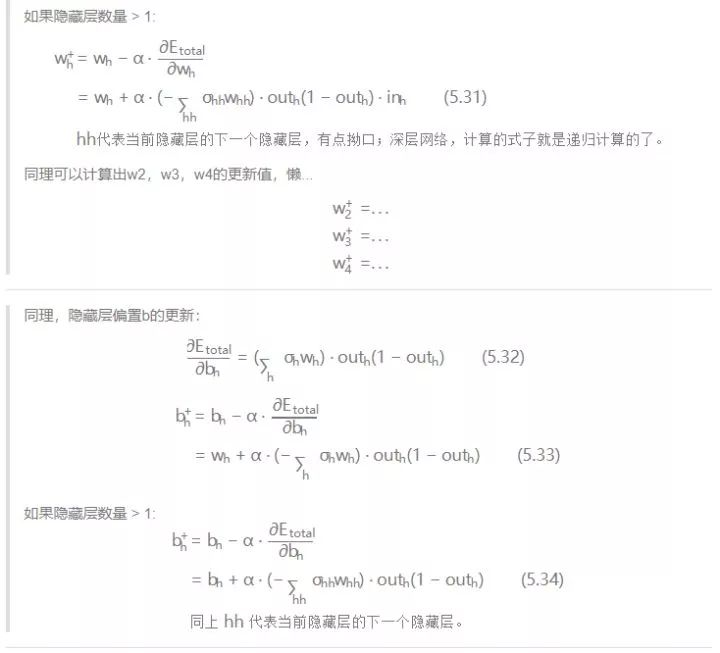## 4.结论：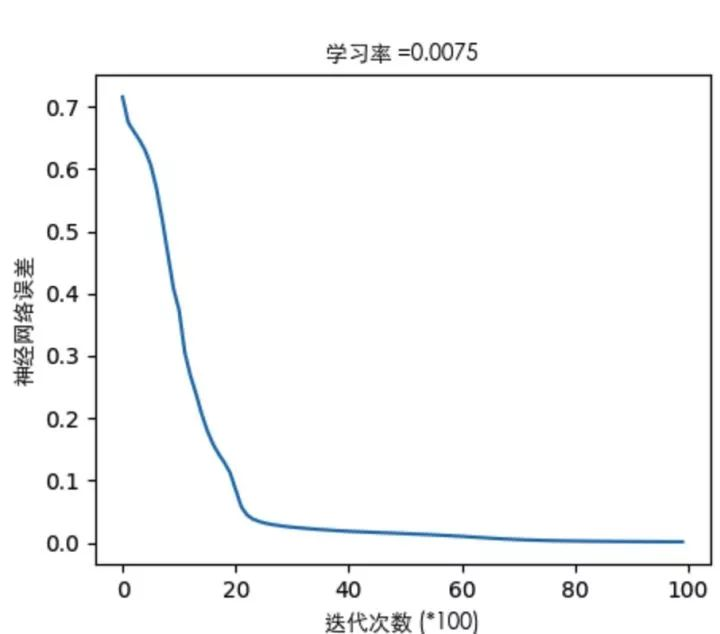## 1. 代码代码！

• 代码实现如下。代码里已经做了尽量啰嗦的注释，关键实现的地方对标了公式的编号，如果看的不明白的地方多回来啃一下算法推导。对应代码也传到了github上。

• 代码能自己定义神经网络的结构，支持深度网络。代码实现了对红蓝颜色的点做分类的模型训练，通过3层网络结构，改变隐藏层的神经元个数，通过图形显示隐藏层神经元数量对问题的解释能力。

• 代码中还实现了不同激活函数。隐藏层可以根据需要换着激活函数玩，输出层一般就用sigmoid，当然想换也随你喜欢～

#coding:utf-8
import h5py
import sklearn.datasets
import sklearn.linear_model
import matplotlib
import matplotlib.font_manager as fm
import matplotlib.pyplot as plt
import numpy as np
np.random.seed(1)
font = fm.FontProperties(fname='/System/Library/Fonts/STHeiti Light.ttc')
matplotlib.rcParams['figure.figsize'] = (10.08.0)
def sigmoid(input_sum):
"""
函数：
激活函数Sigmoid
输入：
input_sum: 输入，即神经元的加权和
返回：
output: 激活后的输出
input_sum: 把输入缓存起来返回
"""

output = 1.0/(1+np.exp(-input_sum))
return output, input_sum
def sigmoid_back_propagation(derror_wrt_output, input_sum):
"""
函数：
误差关于神经元输入的偏导: dE／dIn = dE/dOut * dOut/dIn  参照式（5.6）
其中： dOut/dIn 就是激活函数的导数 dy=y(1 - y)，见式（5.9）
dE/dOut 误差对神经元输出的偏导，见式（5.8）
输入：
derror_wrt_output：误差关于神经元输出的偏导: dE/dyⱼ = 1/2(d(expect_to_output - output)**2/doutput) = -(expect_to_output - output)
input_sum: 输入加权和
返回：
derror_wrt_dinputs: 误差关于输入的偏导，见式（5.13）
"""

output = 1.0/(1 + np.exp(- input_sum))
doutput_wrt_dinput = output * (1 - output)
derror_wrt_dinput =  derror_wrt_output * doutput_wrt_dinput
return derror_wrt_dinput
def relu(input_sum):
"""
函数：
激活函数ReLU
输入：
input_sum: 输入，即神经元的加权和
返回：
outputs: 激活后的输出
input_sum: 把输入缓存起来返回
"""

output = np.maximum(0, input_sum)
return output, input_sum
def relu_back_propagation(derror_wrt_output, input_sum):
"""
函数：
误差关于神经元输入的偏导: dE／dIn = dE/dOut * dOut/dIn
其中： dOut/dIn 就是激活函数的导数
dE/dOut 误差对神经元输出的偏导
输入：
derror_wrt_output：误差关于神经元输出的偏导
input_sum: 输入加权和
返回：
derror_wrt_dinputs: 误差关于输入的偏导
"""

derror_wrt_dinputs = np.array(derror_wrt_output, copy=True)
derror_wrt_dinputs[input_sum <= 0] = 0
return derror_wrt_dinputs
def tanh(input_sum):
"""
函数：
激活函数 tanh
输入：
input_sum: 输入，即神经元的加权和
返回：
output: 激活后的输出
input_sum: 把输入缓存起来返回
"""

output = np.tanh(input_sum)
return output, input_sum
def tanh_back_propagation(derror_wrt_output, input_sum):
"""
函数：
误差关于神经元输入的偏导: dE／dIn = dE/dOut * dOut/dIn
其中： dOut/dIn 就是激活函数的导数 tanh'(x) = 1 - x²
dE/dOut 误差对神经元输出的偏导
输入：
derror_wrt_output：误差关于神经元输出的偏导: dE/dyⱼ = 1/2(d(expect_to_output - output)**2/doutput) = -(expect_to_output - output)
input_sum: 输入加权和
返回：
derror_wrt_dinputs: 误差关于输入的偏导
"""

output = np.tanh(input_sum)
doutput_wrt_dinput = 1 - np.power(output, 2)
derror_wrt_dinput =  derror_wrt_output * doutput_wrt_dinput
return derror_wrt_dinput
def activated(activation_choose, input):
"""把正向激活包装一下"""
if activation_choose == "sigmoid":
return sigmoid(input)
elif activation_choose == "relu":
return relu(input)
elif activation_choose == "tanh":
return tanh(input)
return sigmoid(input)
def activated_back_propagation(activation_choose, derror_wrt_output, output):
"""包装反向激活传播"""
if activation_choose == "sigmoid":
return sigmoid_back_propagation(derror_wrt_output, output)
elif activation_choose == "relu":
return relu_back_propagation(derror_wrt_output, output)
elif activation_choose == "tanh":
return tanh_back_propagation(derror_wrt_output, output)
return sigmoid_back_propagation(derror_wrt_output, output)
class NeuralNetwork:
def __init__(self, layers_strcuture, print_cost = False):
self.layers_strcuture = layers_strcuture
self.layers_num = len(layers_strcuture)
# 除掉输入层的网络层数，因为其他层才是真正的神经元层
self.param_layers_num = self.layers_num - 1
self.learning_rate = 0.0618
self.num_iterations = 2000
self.x = None
self.y = None
self.w = dict()
self.b = dict()
self.costs = []
self.print_cost = print_cost
self.init_w_and_b()
def set_learning_rate(self, learning_rate):
"""设置学习率"""
self.learning_rate = learning_rate
def set_num_iterations(self, num_iterations):
"""设置迭代次数"""
self.num_iterations = num_iterations
def set_xy(self, input, expected_output):
"""设置神经网络的输入和期望的输出"""
self.x = input
self.y = expected_output
def init_w_and_b(self):
"""
函数:
初始化神经网络所有参数
输入:
layers_strcuture: 神经网络的结构，例如[2,4,3,1]，4层结构:
第0层输入层接收2个数据，第1层隐藏层4个神经元，第2层隐藏层3个神经元，第3层输出层1个神经元
返回: 神经网络各层参数的索引表，用来定位权值 wᵢ  和偏置 bᵢ，i为网络层编号
"""

np.random.seed(3)
# 当前神经元层的权值为 n_i x n_(i-1)的矩阵，i为网络层编号，n为下标i代表的网络层的节点个数
# 例如[2,4,3,1]，4层结构：第0层输入层为2，那么第1层隐藏层神经元个数为4
# 那么第1层的权值w是一个 4x2 的矩阵，如：
#    w1 = array([ [-0.96927756, -0.59273074],
#                 [ 0.58227367,  0.45993021],
#                 [-0.02270222,  0.13577601],
#                 [-0.07912066, -1.49802751] ])
# 当前层的偏置一般给0就行，偏置是个1xnᵢ的矩阵，nᵢ为第i层的节点个数，例如第1层为4个节点，那么：
#    b1 = array([ 0.,  0.,  0.,  0.])
for l in range(1, self.layers_num):
self.w["w" + str(l)] = np.random.randn(self.layers_strcuture[l], self.layers_strcuture[l-1])/np.sqrt(self.layers_strcuture[l-1])
self.b["b" + str(l)] = np.zeros((self.layers_strcuture[l], 1))
return self.w, self.b
def layer_activation_forward(self, x, w, b, activation_choose):
"""
函数：
网络层的正向传播
输入：
x: 当前网络层输入（即上一层的输出），一般是所有训练数据，即输入矩阵
w: 当前网络层的权值矩阵
b: 当前网络层的偏置矩阵
activation_choose: 选择激活函数 "sigmoid", "relu", "tanh"
返回:
output: 网络层的激活输出
cache: 缓存该网络层的信息，供后续使用： (x, w, b, input_sum) -> cache
"""

# 对输入求加权和，见式（5.1）
input_sum = np.dot(w, x) + b
# 对输入加权和进行激活输出
output, _ = activated(activation_choose, input_sum)
return output, (x, w, b, input_sum)
def forward_propagation(self, x):
"""
函数:
神经网络的正向传播
输入:
返回:
output: 正向传播完成后的输出层的输出
caches: 正向传播过程中缓存每一个网络层的信息： (x, w, b, input_sum),... -> caches
"""

caches = []
#作为输入层，输出 = 输入
output_prev = x
#第0层为输入层，只负责观察到输入的数据，并不需要处理，正向传播从第1层开始，一直到输出层输出为止
# range(1, n) => [1, 2, ..., n-1]
L = self.param_layers_num
for l in range(1, L):
# 当前网络层的输入来自前一层的输出
input_cur = output_prev
output_prev, cache = self.layer_activation_forward(input_cur, self.w["w"+ str(l)], self.b["b" + str(l)], "tanh")
caches.append(cache)
output, cache = self.layer_activation_forward(output_prev, self.w["w" + str(L)], self.b["b" + str(L)], "sigmoid")
caches.append(cache)
return output, caches
def show_caches(self, caches):
"""显示网络层的缓存参数信息"""
i = 1
for cache in caches:
print("%dtd Layer" % i)
print(" input: %s" % cache)
print(" w: %s" % cache)
print(" b: %s" % cache)
print(" input_sum: %s" % cache)
print("----------")
i += 1
def compute_error(self, output):
"""
函数:
计算档次迭代的输出总误差
输入:
返回:
"""

m = self.y.shape
# 计算误差，见式(5.5): E = Σ1/2(期望输出-实际输出)²
#error = np.sum(0.5 * (self.y - output) ** 2) / m
# 交叉熵作为误差函数
error =  -np.sum(np.multiply(np.log(output),self.y) + np.multiply(np.log(1 - output), 1 - self.y)) / m
error = np.squeeze(error)
return error
def layer_activation_backward(self, derror_wrt_output, cache, activation_choose):
"""
函数:
网络层的反向传播
输入:
derror_wrt_output: 误差关于输出的偏导
cache: 网络层的缓存信息 (x, w, b, input_sum)
activation_choose: 选择激活函数 "sigmoid", "relu", "tanh"
返回: 梯度信息，即
derror_wrt_output_prev: 反向传播到上一层的误差关于输出的梯度
derror_wrt_dw: 误差关于权值的梯度
derror_wrt_db: 误差关于偏置的梯度
"""

input, w, b, input_sum = cache
output_prev = input     # 上一层的输出 = 当前层的输入; 注意是'输入'不是输入的加权和（input_sum）
m = output_prev.shape      # m是输入的样本数量，我们要取均值，所以下面的求值要除以m
# 实现式（5.13）-> 误差关于权值w的偏导数
derror_wrt_dinput = activated_back_propagation(activation_choose, derror_wrt_output, input_sum)
derror_wrt_dw = np.dot(derror_wrt_dinput, output_prev.T) / m
# 实现式 （5.32）-> 误差关于偏置b的偏导数
derror_wrt_db = np.sum(derror_wrt_dinput, axis=1, keepdims=True)/m
# 为反向传播到上一层提供误差传递，见式（5.28）的 （Σδ·w） 部分
derror_wrt_output_prev = np.dot(w.T, derror_wrt_dinput)
return derror_wrt_output_prev, derror_wrt_dw, derror_wrt_db
def back_propagation(self, output, caches):
"""
函数:
神经网络的反向传播
输入:
output：神经网络输
caches：所有网络层（输入层不算）的缓存参数信息  [(x, w, b, input_sum), ...]
返回:
"""

L = self.param_layers_num #
output = output.reshape(output.shape)  # 把输出层输出输出重构成和期望输出一样的结构
expected_output = self.y
# 见式(5.8)
#derror_wrt_output = -(expected_output - output)
# 交叉熵作为误差函数
derror_wrt_output = - (np.divide(expected_output, output) - np.divide(1 - expected_output, 1 - output))
# 反向传播：输出层 -> 隐藏层，得到梯度：见式(5.8), (5.13), (5.15)
current_cache = caches[L - 1# 取最后一层,即输出层的参数信息
self.layer_activation_backward(derror_wrt_output, current_cache, "sigmoid")
# 反向传播：隐藏层 -> 隐藏层，得到梯度：见式 (5.28)的(Σδ·w), (5.28), (5.32)
for l in reversed(range(L - 1)):
current_cache = caches[l]
derror_wrt_output_prev_temp, derror_wrt_dw_temp, derror_wrt_db_temp = \
self.layer_activation_backward(grads["derror_wrt_output" + str(l + 2)], current_cache, "tanh")
grads["derror_wrt_output" + str(l + 1)] = derror_wrt_output_prev_temp
grads["derror_wrt_dw" + str(l + 1)] = derror_wrt_dw_temp
grads["derror_wrt_db" + str(l + 1)] = derror_wrt_db_temp
"""
函数:
根据梯度信息更新w，b
输入:
返回:
"""

# 权值w和偏置b的更新，见式:（5.16),(5.18)
for l in range(self.param_layers_num):
self.w["w" + str(l + 1)] = self.w["w" + str(l + 1)] - self.learning_rate * grads["derror_wrt_dw" + str(l + 1)]
self.b["b" + str(l + 1)] = self.b["b" + str(l + 1)] - self.learning_rate * grads["derror_wrt_db" + str(l + 1)]
def training_modle(self):
"""训练神经网络模型"""
np.random.seed(5)
for i in range(0, self.num_iterations):
# 正向传播，得到网络输出，以及每一层的参数信息
output, caches = self.forward_propagation(self.x)
# 计算网络输出误差
cost = self.compute_error(output)
# 反向传播，得到梯度信息
# 根据梯度信息，更新权值w和偏置b
# 当次迭代结束，打印误差信息
if self.print_cost and i % 1000 == 0:
print ("Cost after iteration %i: %f" % (i, cost))
if self.print_cost and i % 1000 == 0:
self.costs.append(cost)
# 模型训练完后显示误差曲线
if False:
plt.plot(np.squeeze(self.costs))
plt.ylabel(u'神经网络误差', fontproperties = font)
plt.xlabel(u'迭代次数 (*100)', fontproperties = font)
plt.title(u"学习率 =" + str(self.learning_rate), fontproperties = font)
plt.show()
return self.w, self.b
def predict_by_modle(self, x):
"""使用训练好的模型（即最后求得w，b参数）来决策输入的样本的结果"""
output, _ = self.forward_propagation(x.T)
output = output.T
result = output / np.sum(output, axis=1, keepdims=True)
return np.argmax(result, axis=1)
def plot_decision_boundary(xy, colors, pred_func):
# xy是坐标点的集合，把集合的范围算出来
# 加减0.5相当于扩大画布的范围，不然画出来的图坐标点会落在图的边缘，逼死强迫症患者
x_min, x_max = xy[:, 0].min() - 0.5, xy[:, 0].max() + 0.5
y_min, y_max = xy[:, 1].min() - 0.5, xy[:, 1].max() + 0.5
# 以h为分辨率，生成采样点的网格，就像一张网覆盖所有颜色点
h = .01
xx, yy = np.meshgrid(np.arange(x_min, x_max, h), np.arange(y_min, y_max, h))
# 把网格点集合作为输入到模型，也就是预测这个采样点是什么颜色的点，从而得到一个决策面
Z = pred_func(np.c_[xx.ravel(), yy.ravel()])
Z = Z.reshape(xx.shape)
# 利用等高线，把预测的结果画出来，效果上就是画出红蓝点的分界线
plt.contourf(xx, yy, Z, cmap=plt.cm.Spectral)
# 训练用的红蓝点点也画出来
plt.scatter(xy[:, 0], xy[:, 1], c=colors, marker='o', cmap=plt.cm.Spectral, edgecolors='black')
if __name__ == "__main__":
plt.figure(figsize=(1632))
# 用sklearn的数据样本集，产生2种颜色的坐标点，noise是噪声系数，噪声越大，2种颜色的点分布越凌乱
xy, colors = sklearn.datasets.make_moons(60, noise=1.0)
# 因为点的颜色是1bit，我们设计一个神经网络，输出层有2个神经元。
# 标定输出[1,0]为红色点，输出[0,1]为蓝色点
expect_output = []
for c in colors:
if c == 1:
expect_output.append([0,1])
else:
expect_output.append([1,0])
expect_output = np.array(expect_output).T
# 设计3层网络，改变隐藏层神经元的个数，观察神经网络分类红蓝点的效果
hidden_layer_neuron_num_list = [1,2,4,10,20,50]
for i, hidden_layer_neuron_num in enumerate(hidden_layer_neuron_num_list):
plt.subplot(52, i + 1)
plt.title(u'隐藏层神经元数量: %d' % hidden_layer_neuron_num, fontproperties = font)
nn = NeuralNetwork([2, hidden_layer_neuron_num, 2], True)
# 输出和输入层都是2个节点，所以输入和输出的数据集合都要是 nx2的矩阵
nn.set_xy(xy.T, expect_output)
nn.set_num_iterations(30000)
nn.set_learning_rate(0.1)
w, b = nn.training_modle()
plot_decision_boundary(xy, colors, nn.predict_by_modle)
plt.show()

## 2. 晒图晒图！

• 通过看误差曲线，可以从一定程度上判定网络的效果，模型训练是否能收敛，收敛程度如何，都可以从误差曲线对梯度下降的过程能见一二。3层网络的结构下，隐藏层只有一层，看图说明一下隐藏层神经元个数变化对神经网络表达能力的影响：

• 当隐藏层只有1个神经元：就像文章刚开始说的，一个神经元，就是个线性分类器，表达能力就一条直线而已，见式（3.6）

• 2个神经元：线开始有点弯曲了，但是这次结果一点都不明显，尴尬。但从原理上神经网络开始具备了非线性表达能力

• 随着隐藏层神经元个数不断增加，神经网络表达能力越来越强，分类的效果越来越好。当然也不是神经元越多越好，可以开始考虑深度网络是不是效果更好一些。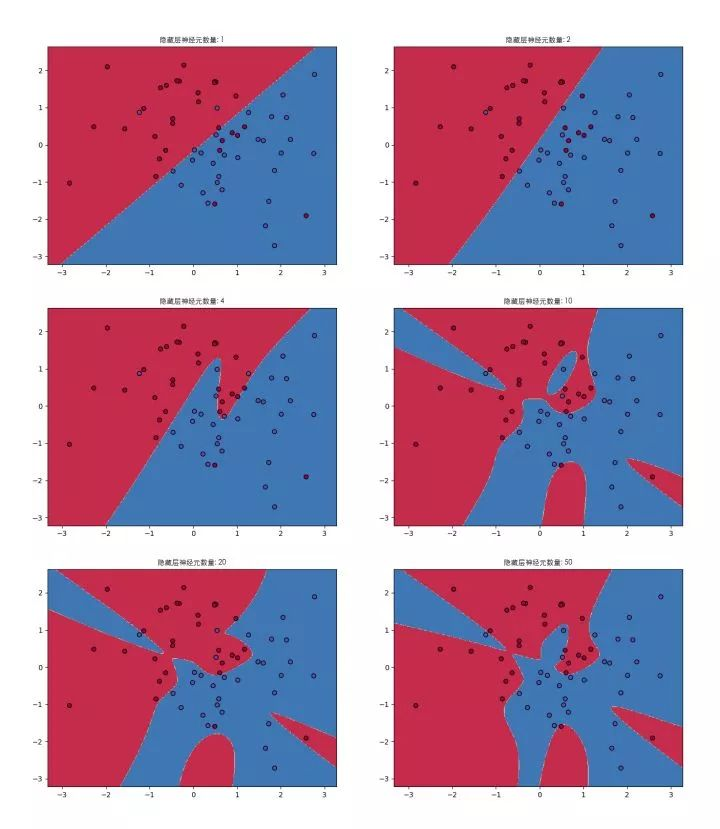## ▌7. 没有结局

• 神经网络的结构，即几层网络，输入输出怎么设计才最有效？

• 数学理论证明，三层的神经网络就能够以任意精度逼近任何非线性连续函数。那么为什么还需要有深度网络？

• 在不同应用场合下，激活函数怎么选择？

• 学习率怎么怎么选择？

• 训练次数设定多少训练出的模型效果更好？

AI，从入门到放弃，首篇结束。

https://zhuanlan.zhihu.com/p/38006693

AI公开课04-2001-28
04-197683
07-164616
01-051万+
03-282318
11-043万+
05-123134
11-27634
03-012737
05-312万+
03-112万+
04-08
06-03
11-175万+
10-25244
11-16888
05-262347点击重新获取扫码支付1.余额是钱包充值的虚拟货币，按照1:1的比例进行支付金额的抵扣。
2.余额无法直接购买下载，可以购买VIP、C币套餐、付费专栏及课程。余额充值